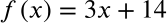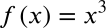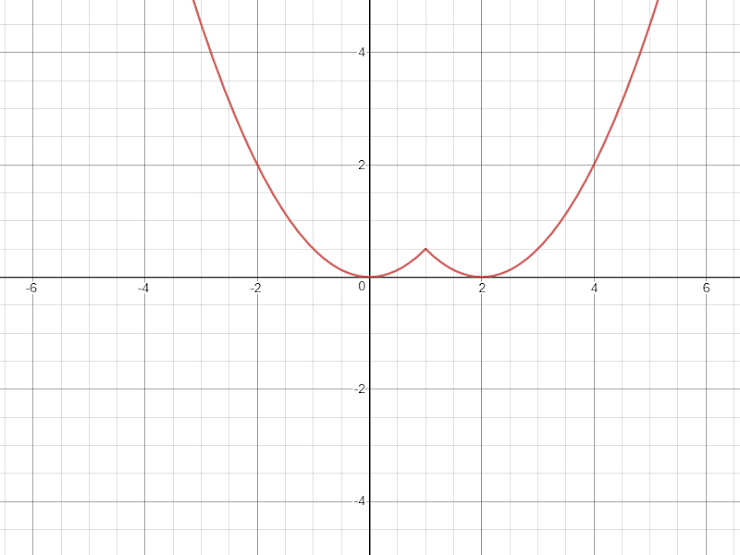Exit Ticket for Day 14 in Calculus
Please select your name from the following list: *
1. For the following function, find its derivative, f'(x):2. For the following function, find its derivative, f'(x):3. Does the function shown below have a derivative at x=-2?4. Using the same graph as in the previous question, does the function shown have a derivative at x=1?
5. Is the function graphed in problem 3 "continuous"?
6. Suppose you have a function that describes the mass of a raindrop as a function of the radius of the raindrop (assuming that the raindrops are spherical). Interpret the DERIVATIVE of that function.
This form was created inside of Evansville Vanderburgh School Corporation. Report Abuse - Terms of Service